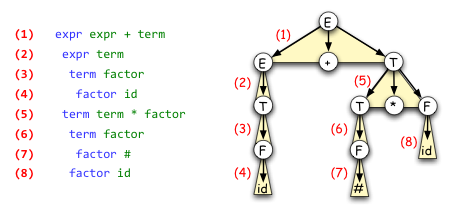CS 241 - CFG File Format (.cfg)

A .cfg file is a text file representing a context-free grammar followed by several (zero or more) abbreviated leftmost derivations.

Context-free Grammar Representation

The context-free grammar representation has four components, in order:
• t, a positive integer giving the number of terminal symbols in the grammar, followed by t lines, each containing the name of a distinct terminal symbol. Each terminal symbol may be any string of one or more printable ascii characters, excluding white space.
• n, a positive integer giving the number of nonterminal symbols in the grammar, followed by n lines, each containing the name of a distinct nonterminal symbol. Each nonterminal symbol may be any string of one or more printable ascii characters, and must not be the same as any terminal symbol.
• A single line giving the name of the start symbol, which must be one of the nonterminal symbols
• r, a positive integer giving the number of production rules in the grammar, followed by r lines, each denoting a rule consisting of:
• The left-hand-side (LHS) of the rule; a nonterminal symbol
• The right-hand-side (RHS) of the rule; zero or more terminal or nonterminal symbols separated from each other, and from the LHS by a single space

Abbreviated Leftmost Derivation(s)

Zero or more derivations immediately follow the context-free grammar. Each derivation is a representation of the parse tree, with each node in the tree represented by a line in the file containing a production rule indented exactly one space to the right of its parent. (That is, the number of spaces of indentation is the same as the depth of the node in the tree.)

The order and indentation of the lines in the file are defined by the following recursive rules:

• Each line in the file is a production rule indented k spaces.
• The first line of the file is a production rule whose LHS is the start symbol, indented 0 spaces. This line represents the root of the parse tree.
• Following each production rule that is indented k spaces and has n nonterminal symbols in its RHS, are n sets of lines, each set representing the subtree corresponding to one of the nonterminal symbols, in the order they appear in the rule.
• The subtree for each nonterminal begins with a rule, indented k+1 spaces, whose LHS is the corresponding nonterminal. The remaining lines in the set are determined by applying this rule recursively.

Here, for example, is an illustration of the correspondence between the lines in an abbreviated leftmost derivation and the corresponding derivation tree.cs241.cfgcheck Tool

The student.cs environment includes a tool "CFGCheck" that verifies the contents of a .cfg file. If the CFG and derivations are valid, it prints the CFG and the rules expanded in leftmost order. It then prints the terminal string arrived at by the derivation.

If the CFG is malformed, or the derivation is invalid, an error message is printed, and the tool quits.

To use the tool:

cs241.cfgcheck < sample.cfg

Example .cfg file (sample.cfg)

6
BOF
EOF
id
-
(
)
3
S
expr
term
S
5
S BOF expr EOF
expr term
expr expr - term
term id
term ( expr )
S BOF expr EOF
expr expr - term
expr expr - term
expr term
term id
term ( expr )
expr term
term id
term id

Output of cs241.CFGCheck on sample.cfg

Terminals:
BOF
EOF
id
-
(
)

Nonterminals:
S
expr
term

Start Symbol:
S

Production Rules:
S BOF expr EOF
expr term
expr expr - term
term id
term ( expr )

Derivation Steps:
S ->  BOF expr EOF
expr ->  expr - term
expr ->  expr - term
expr ->  term
term ->  id
term ->  ( expr )
expr ->  term
term ->  id
term ->  id

Terminal String:
BOF id - ( id ) - id EOF